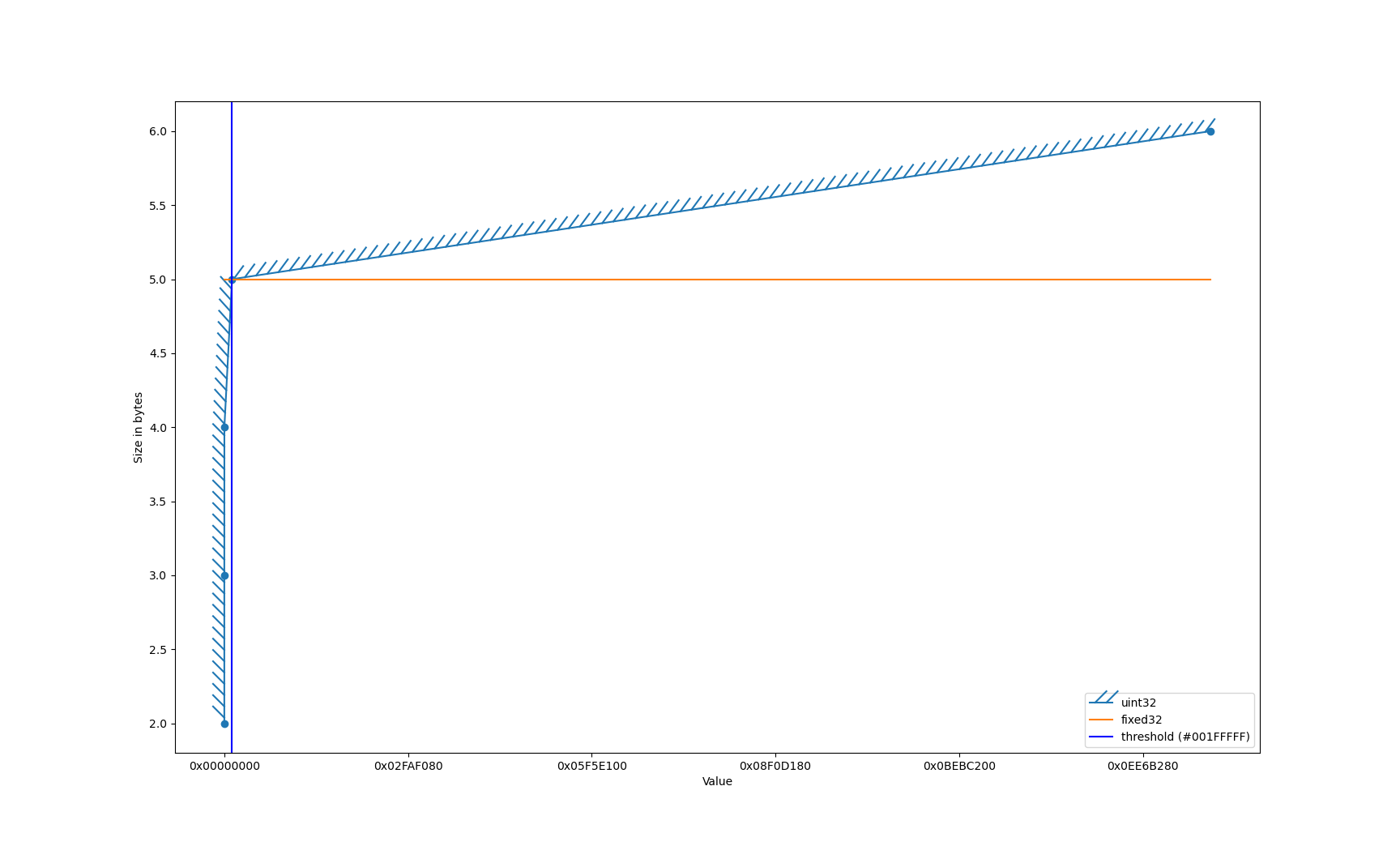# Storing Colors in Protocol Buffers

While working on a new course, I was looking for an example to store a Color in Protocol Buffers. At first this seemed like an easy task but it turned out to be an interesting example of optimization. Let's work through it.

## Quick Requirements

In order to define what's the most optimal message definition that we come with, we need a way to calculate the serialized size of that message. Fortunately, doing so is pretty easy with Protocol Buffers.

• Copied!
``````def calculate_size(message):
return len(message.SerializeToString())
``````
• ``````import com.google.protobuf.Message;

int calculateSize(Message message) {
return message.getSerializedSize();
}
``````
• ``````import com.google.protobuf.Message

fun calculateSize(message: Message) = message.serializedSize
``````
• ``````import "google.golang.org/protobuf/proto"

func calculateSize(message proto.Message) int {
out, err := proto.Marshal(message)

if err != nil {
log.Fatalln("Failed to encode:", err)
}

return len(out)
}
``````
• ``````using Google.Protobuf

int CalculateSize(IMessage message) {
return message.CalculateSize();
}
``````
• ``````function calculateSize(message) {
return message.serializeBinary().length;
}
``````
• ``````#include <google/protobuf/message.h>

{
std::string out;
bool serialized = message->SerializeToString(&out);

if (!serialized) {
return -1;
}

return out.length();
}
``````

## A primitive implementation

When I see something like `#FFFFFFFF` or `#00000000` (RGBA), I directly think about two things:

• The human readable solution: `string`
• The non human readable solution: `int32` or `int64`

Let's try with the string and work our way through, here is the proto file we are gonna use:

``````syntax = "proto3";

option java_package = "com.example";
option java_multiple_files = true;
option go_package = "example.com/m";
option csharp_namespace = "Example";

message Color {
string value = 1;
}
``````

and here is the code that calculates the size for `Color` with value `#FFFFFFFF` (max color value):

• ``````import proto.color_pb2 as pb

print(calculate_size(pb.Color(value = "FFFFFFFF")))
``````
• ``````import com.example.Color

System.out.println(calculateSize(Color.newBuilder().setValue("FFFFFFFF").build()));
``````
• ``````import com.example.color

println(calculateSize(color { value = "FFFFFFFF" }))
``````
• ``````import pb "example.com/m"

fmt.Println(calculateSize(&pb.Color{Value: "FFFFFFFF"}))
``````
• ``````using Example;

Console.WriteLine(CalculateSize(new Color { Value = "FFFFFFFF" }));
``````
• ``````const {Color} = require('./proto/color_pb');

console.log(calculateSize(new Color().setValue("FFFFFFFF")));
``````
• ``````#include "color.pb.h"

Color color;

color.set_value("FFFFFFFF");
std::cout << calculate_size(color) << std::endl;
``````

And that should give us a 10 bytes serialization, because this will be encoded as the following:

0a 08 46 46 46 46 46 46 46 46

where:

🔵 blue: is the combinaison between field tag and field type in one byte (read more here). In our case our tag is 1 and the type is what's called `Length-delimited`.

🔴 red: is the size of the `Length-delimited` field, here 8.

🟢 green: is the `Length-delimited` field value. Here 46 is F (you can type `man ascii` and have a look at the Hexadecimal set).

## Let's optimize that

As mentioned earlier, the other way to solve that is to store the value in an integer. So let's check the decimal value of the biggest color that we can get, which is `FFFFFFFF`.

• ``````echo "ibase=16; FFFFFFFF" | bc
``````
• ``````[convert]::toint64("FFFFFFFF", 16)
``````

and this gives us: 4,294,967,295. Sounds like this gonna fit inside an `int32` or even an `uint32` if we wanted to make class instantiation safer (not letting user enter negative value). So we now have:

``````message Color {
uint32 value = 1;
}
``````

and by using the same code for calculating the size we obtain: 6 bytes.

## A step further

Let's take a look at a table that I made for another post.

Threshold value Bytes size (without tag)
0 0
1 1
128 2
16,384 3
2,097,152 4
268,435,456 5

This table presents the field value thresholds and the bytes size for serialization of `uint32`. Can you see the problem here ? 4,294,967,295 is simply bigger than 268,435,456 and what it means is that, our value of `FFFFFFFF` will be serialized to 5 bytes.

Do we know another type that could help us serialize in less bytes? Sure we do! We know that `fixed32` is an unsigned integer and it will always be serialized to 4 bytes. So we if change to:

``````message Color {
fixed32 value = 1;
}
``````

the value `FFFFFFFF` will be serialized into:

0d ff ff ff ff

and we are done!

## Wait a minute ...

This seems to vary with our data/color distribution, isn't it ?It varies. However you can see the number of colors that can be efficiently serialized with a `uint32` is pretty small. The dots here represent the threshold that I showed in the table presented in "A step further" and here we can see that the threshold at 2,097,152 or `001FFFFF` is where it becomes efficient to store with a `fixed32`.

Let's calculate the percentage of colors that can be efficiently stored with an `uint32`.

(2097152 / 4294967295) * 100 ~= 0.05

where:

🔵 blue: is the threshold at which it becomes more optimal to save with `fixed32`.

🔴 red: biggest number that we can have (`FFFFFFFF`).

So in conclusion only 0.05% of the possible numbers will be not optimally serialized. I think we can agree on the fact that is acceptable.

## Conclusion

Protocol Buffers are providing us with a lot of types for numbers, and choosing the right one is important for optimizing you payload or serialized data size. If you want to know more about how to choose between them, you might consider joining my Udemy course on Protocol Buffers.

Hope you enjoyed this article, I will be glad to get some feedback on this. Especially if you find a more efficient way to serialize this data. Check the about page to find all the ways you can us for reaching to me.

Written on June 2, 2022